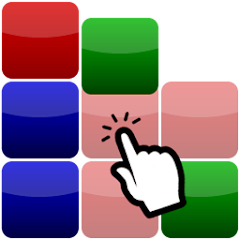# Block Blast

1K+
Teacher ApprovedEveryone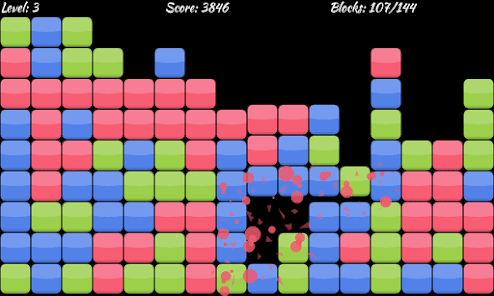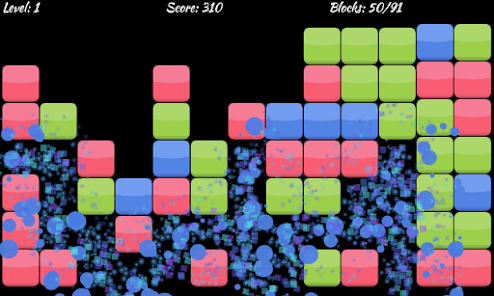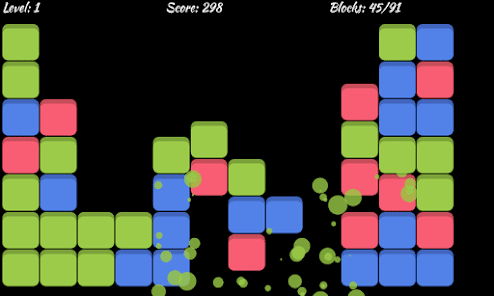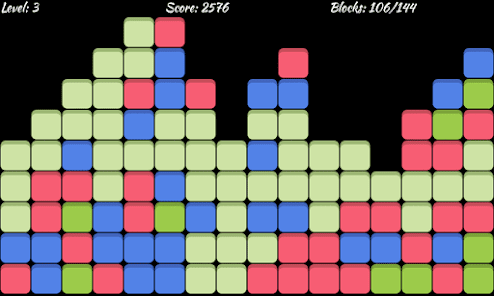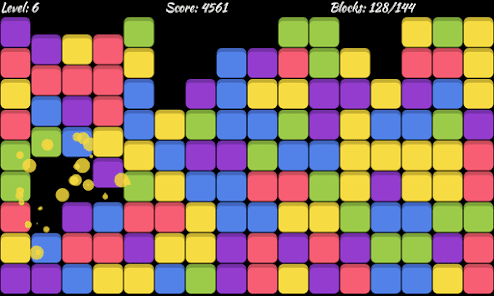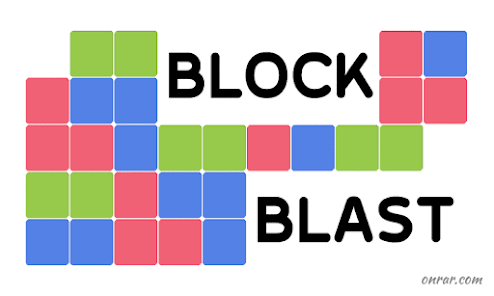Block Blast is a puzzle game. You have to blast adjacent same-colored blocks to complete the board. Blasting larger blocks, you will gain more points. In addition, try to decrease the number of remaining un-blasted blocks to gain better level bonus. If you have more than 10 remaining blocks at the end of level, the game is over for you. Here are the explanations of the game:

========= EXPLANATION 1 =========

Try to explode larger blocks to gain better scores.
To this end, firstly focus on interstitial blocks to produce larger adjacent blocks having the same color.

Blast Award = (N + L) x (N + L - 1) / 2
N: number of adjacent blocks, L: level

========= EXPLANATION 2 =========

Try to reduce the number of remaining blocks.
To this end, be careful at the last explosions.
Clear interstitial blocks to join separated colors.

Ending Award = (C - 2) * 1000 / (R + 1)
R: number of remaining blocks, C: number of colors

========= EXPLANATION 3 =========

Game over limit for each level is 10 blocks.
You need to be careful about remaining un-blasted blocks.
If the number of remaining blocks at the end of the level is larger than 10, the game will be over for you...

========= OTHER DETAILS =========

There are 6 levels in the game. Here are the details of these levels:

- Level 1: 3 colors, 13 x 7 = 91 blocks
- Level 2: 3 colors, 14 x 8 = 112 blocks
- Level 3: 3 colors, 16 x 9 = 144 blocks
- Level 4: 4 colors, 14 x 8 = 112 blocks
- Level 5: 4 colors, 16 x 9 = 144 blocks
- Level 6: 5 colors, 16 x 9 = 144 blocks

Blast awards of the levels:

- Level 1: N * (N + 1) / 2
- Level 2: (N + 1) * (N + 2) / 2
- Level 3: (N + 2) * (N + 3) / 2
- Level 4: (N + 3) * (N + 4) / 2
- Level 5: (N + 4) * (N + 5) / 2
- Level 6: (N + 5) * (N + 6) / 2

where N is the number of blasted blocks.

Ending awards of the levels:

- Level 1: 1000 / (R + 1)
- Level 2: 1000 / (R + 1)
- Level 3: 1000 / (R + 1)
- Level 4: 2000 / (R + 1)
- Level 5: 2000 / (R + 1)
- Level 6: 3000 / (R + 1)

where R is the number of remaining un-blasted blocks at the level end.
Updated on
Feb 14, 2020

## Data safety